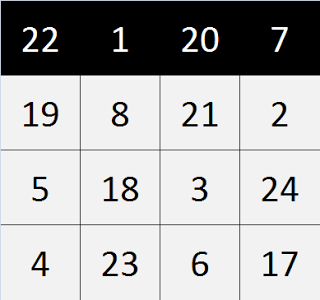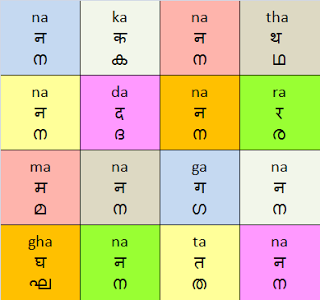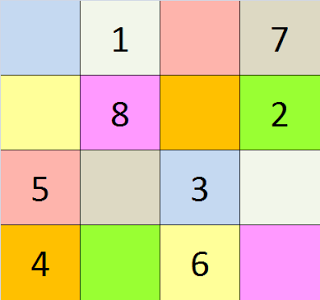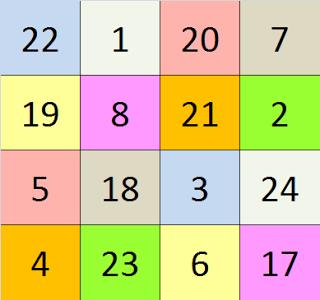The Katapayadi system of representing numbers that we introduced in a previous post opens up the possibility of numerous tricks and techniques to memorize numbers and patterns of numbers that are useful, or otherwise interesting. An example of this is an easy technique involving this number system to create ultimate magic squares with perfect symmetry. With the breed of journalism that we are exposed to these days you are justified in thinking that the words ultimate and perfect might have been used only to sensationalize and create an air of importance; but I urge you to read up to the end and decide for yourself if the use of these qualifiers are justified.
This technique, which I learnt from my grandmother, is a step-by-step method for creating 4×4 magic squares with a desired sum. For example, let us say I want a magic square with sum as 50. Any magic square that is worth that name would ensure that I have a 4×4 matrix with the rows and columns each adding up to the same number – 50 in this case. This condition is easily satisfied in the one generated using our techniqueExample of row sum (50)Example of column sum (50)
The reason we call ours an ultimate magic square and take pride in its perfect symmetry is because it satisfies many other conditions. To start with, the sums of the two diagonals also each equal our desired sumExample of diagonal sum (50)
It just gets better from here. Take the sum of digits in cells forming any of the 2×2 squares, and again you get the same desired sumExample of 2×2 square sum (50)

What’s more, even larger squares within this (3×3 and 4×4) have the sum of their corner cells equal to the desired sum.Corner cells sum (50)Example of 3×3 squares’ corner cells sum (50)

Isn’t the symmetry of this magic square in the superlative? What is more amazing is that the technique with which it has been created can be generalized to generate magic squares of any desired sum.

We start with an empty 4×4 square and fill its cells based on the rules given by the following sloka:

नाकनाथ नदीनीरे
मानाङ्गिनि घनस्तनि
इष्टार्धे एकशून्ये
गजपादक्रमात् लिखेत्
നാകനാഥ നദീനീരെ
മാനാങ്ഗിനി ഘനസ്തനി
ഇഷ്ടാര്ധേ ഏകശൂന്യേ
ഗജപാദക്രമാത് ലിഖേത്
First step is to fill each cell with the letters from the first two lines of the sloka, which gives us the square shown below:Step 1: Populate 4×4 square with letters from first two lines of the sloka
Next, replace the letters with their value based on Katapayadi system. Cells which have a value of zero may be left blank.Step 2: Replace letters with their Katapayadi values
To fill out the remaining cells, we need to follow the rule specified by the third and fourth lines of the shloka, which may roughly be translated as “Starting from one less than half of the desired sum, write in the order in which Elephant’s movement is constrained on the board of Chathurangam”. In Chathurangam, which is the original form of the game of Chess, the Elephant plays the role of Bishop in modern Chess. While the bishop can move to any cell along in its diagonal direction, Elephant can move only to the third cell (with the present cell being the first cell) in the diagonal direction. If our magic-square-in-the-making were to be part of a board of Chathurangam and the numbers marked positions of elephant, the other cell with the same colour will be the only position on this part of the board to which the elephant will be able to move.
To illustrate the rule with an example, let us use 50 as our desired sum, half of which is 25. Starting with 24 (one less than 25) and writing it in the cell with the same colour as 1, we continue to write one less than the previously written number into the cell with the same colour as the one marked by the next smallest of the first eight natural numbers. The first two of these are shown in the figures belowStep 3: Fill in the third square diagonally from 1Step 4: Fill in the third square diagonally form 2
Following this procedure, we end up with the completely filled magic square that we started our discussion with.Step 10: Fill in the last remaining cell to complete the magic square
It can be seen that this technique gives the general idea in symmetrically populating the grid, and that multiplying all values in the magic square with a number will still give a magic square with the desired sum also being multiplied as many times. (Yes, this is the effect of multiplication being distributed over addition). Another point to note is that we can work with negative numbers and floating points as well to some extent, but the square might lose some of its original charm that comes from its neatness and simplicity. For the same reason, it is best to keep the desired sum as even, so that we don’t have to deal with the decimal point. Finally, we note that the Katapayadi system conventionally represents numbers from the unit’s place to higher powers of 10. Since we are used to writing these from right to left, we’re used to seeing Katapayadi words being read from right to left. If this convention is followed, we’ll still have pretty much the same magic square, except that it would be laterally inverted.
If you notice any other interesting property of this technique or the squares it generates, or if you have smart ideas such as extending it to the creation of larger or smaller magic squares, you are encouraged to share those thoughts as comments for the benefit of a larger audience.
• 19
•
•
•
•
•
•
•
•
•
•
19
Shares

## 2 thoughts on “Magic Squares With Katapayadi”

1.Reji says:

This is amazing. But this technique, can this be used for a square matrix of any other order? Like 5×5 or 6×6 etc. Or specific only to 4×4?

1.raman says:

I don't think there's a straight-forward way of extending this technique to a general square matrix, though there could be similar techniques that are applicable there. One possibility of extending this is to an 8×8 square, since it consists of four 4×4 squares, each of which can be created with half of the desired total sum. This would make it a magic square of our desired sum, but not sure if all the other types of symmetry will be exhibited by the larger square.